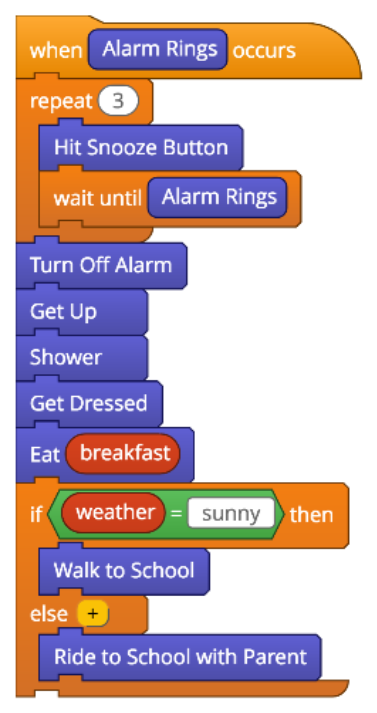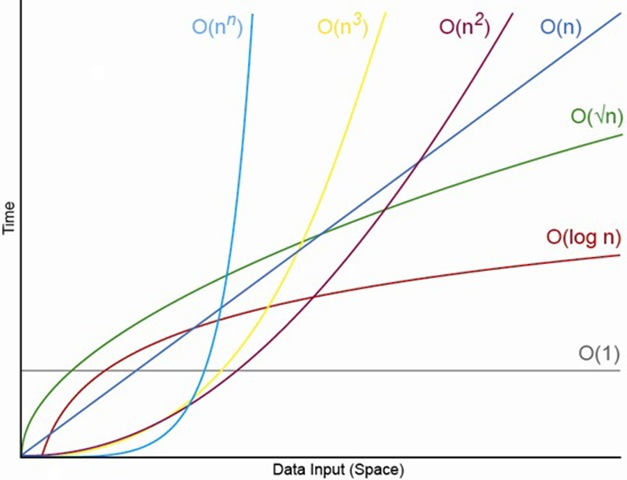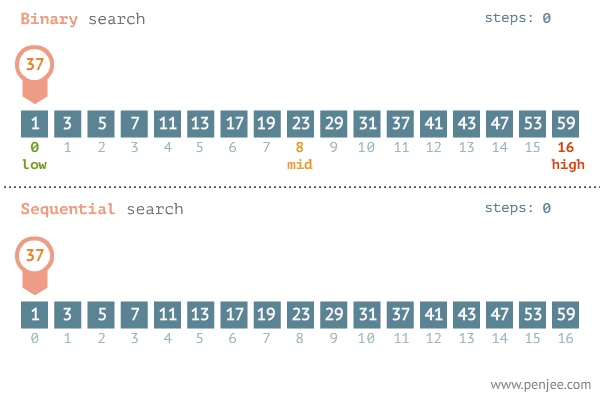# A Brief Introduction to Algorithms and Big-O Notation

Algorithms are a fundamental part of programming, computer science, and often, job interviews. As beginner programmers, algorithms can be daunting, but whether we realize it or not we’re already implementing them in different ways through the decisions we make in our code.

### What are algorithms?

An algorithm is any repeatable process for finding the solution to a problem. If you can outline how to get to the solution step-by-step, and if you can replicate those results, you are probably using an algorithm.

### Algorithms in every day life

Algorithms don’t have to be scary - there are many algorithms at play in every-day life. Some basic algorithms include:

• A recipe for baking cookies
• Reorganizing your closet
• Your morning routine### Why do algorithms matter?

There are many algorithms, and each one has a different impact on how long your code takes to run, and how much memory it takes to run. That may not make a huge difference for our code today, but as our applications grow bigger and more complex it becomes increasingly important to assure that we’re writing code that’s efficient - that is, code that’s fast and does not take up a lot of space.

You can imagine that customers expect the websites, mobile apps, and other applications to load fast and not eat up tons of memory.

### Algorithm efficiency and space/time complexity

Algorithm efficiency is measured in time and space complexity. Time complexity looks at how long an algorithm takes to solve, and space complexity is concerned with how much memory the algorithm takes to run. We usually end up talking more about time complexity, since space is usually more readily available than patience.

Let’s tackle time complexity through a mathematical framework called Big O notation:In simple terms, according to Simple English Wikipedia, “Big O Notation is a way of saying how much time it takes for a mathematical algorithm to run or how much memory it uses.”

### Three examples and their Big O time complexity:

Example 1: Look up an element in an array:

In the above example, the Big O time complexity is O(1) constant in that it takes the same amount of time regardless of input size. If the array was twice the size, the time it takes to run would be the same since we are always looking for the second element in the array.

Example 2: Iterate over an array:

In the above example, the Big O time complexity is O(n) linear in that the time it takes corresponds to the size of the input. If the array was twice the size, the time it takes to run also grows, so it would take twice as long.

Example 3: Binary search on a sorted array:In the above example, the Big O time complexity is O(log n) logarithmic in that the time it takes is divided by half each time. In this case, the algorithm is actually more efficient over time since the size of the array we have to search is cut in half each time.

There are many more Big O notation complexities, and tons of algorithms. A handy tool for Big O is the Big O Cheat Sheet, linked below.

### Reference

Big O Notation, Simple English Wikipedia
https://simple.wikipedia.org/wiki/Big_O_notation

Big O Cheat Sheet
http://bigocheatsheet.com/

Girl Develop It, Intro to Algorithms
http://www.teaching-materials.org/algorithms/#/

CodeNewbie, Episode 103: Algorithms
http://www.codenewbie.org/podcast/algorithms

Cracking the Coding Interview, Gayle Laakmann McDowell
https://www.careercup.com/book

The Algorithm Design Manual, Steven Skiena
http://www.algorist.com/

Introduction to Algorithms, CLRS
https://mitpress.mit.edu/books/introduction-algorithms